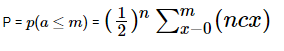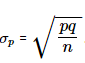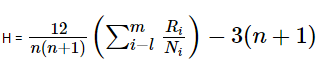# Non-Parametric Test

Non-parametric tests are experiments which do not require the underlying population for assumptions. It does not rely on any data referring to any particular parametric group of probability distributions. Non-parametric methods are also called distribution-free tests since they do not have any underlying population.

## Definition

Non-parametric tests are the mathematical methods used in statistical hypothesis testing, which do not make assumptions about the frequency distribution of variables that are to be evaluated. The non-parametric experiment is used when there are skewed data and it comprises techniques that do not depend on data pertaining to any particular distribution.

The word non-parametric does not mean that these models do not have any parameters. The fact is, the characteristics and number of parameters are pretty flexible and not predefined. Therefore, these models are called distribution-free models.

### Applications of Non-Parametric Test

The conditions when non-parametric tests are used are listed below:

• When parametric tests are not satisfied.
• When testing hypothesis does not have any distribution.
• For quick data analysis.
• When unscaled data is available.

 Advantages Disadvantages Easily understandable Short calculations Assumption of distribution is not required Applicable to all types of data Less efficient as compared to parametric test The results may or may not provide an accurate answer because they are distribution free.

## Sign Test Statistics

The sign test is conducted under the following conditions.

1. When we need to compare paired data
2. The paired data obtained from similar conditions

Sign Test is merely based on the signs (+ or -) of the deviations x-y and not on their magnitudes. This test is applicable when zero differences or tie between the paired observations cannot occur. If zero differences or tie occur, then they will be eliminated from the analysis and the number of paired observations counted is reduced. This method can be used to examine individual data also.

### Sign Test Assumption

Let (x1y1),(x2,y2),….,(xn,yn) be paired observations and di = xi-yi are the differences between the observations, where i=1,2,….,n.

• The value of di can be positive or negative and all those values are independent.
• Each di comes from the same continuous population.
• The values xi and yi represent the order, so that the comparisons “greater than”, “less than”, and “equal to” are meaningful.

Now we will take here the Null hypothesis, H0 : p=½ = 0.5 and Alternate hypothesis, H1:q ≠ ½=0.5.

Let us consider the number of positive signs in di be m. Therefore,

p = m/n and q = n – (m/n)

Case 1(n < 30)

If either np or nq is less than 5, then we can use binomial approximation.where m is the number of positive deviations.

If P > α then we accept the null hypothesis else we reject it.

Case 2 (n < 30):

If both np or nq > 5, then we use a normal distribution for approximation.The limits of the approval region are given by (p-zpσp,p+zpσp), where zp is the value obtained from the standard normal table with α level of significance. If the value of α is not given, we consider it as, α = 0.05.

If ‘p’ lies within (p-zpσp,p+zpσp) we accept the hypothesis, else we reject it.

Case 3 (n ≥ 30)

If n ≥ 30, then we can find Mean = np and standard deviation = npq

And, a = number of negative observations

## Kruskal-Wallis H-test

Kruskal Wallis H test is used to test whether two or more populations are identical. In this test, the null hypothesis is H01 = μ2 = γ3 (when there are three populations) and the alternative hypothesis is H1:μ1≠μ2≠γ3. In the Kruskal-Wallis test, we first evaluate the ranks of the observation lists in the samples and then determine the rank sums for each sample. To calculate the test result, we use the below formula:where,

n is the total number of observations in all samples,

m is the number of samples,

ni represents the number of observations in ith sample,

Ri denotes the rank sum of ith sample.

Here, we have to use the x2 distribution with m – 1, degree of freedom (df) and α level of significance to determine the critical value. If the estimated value is less than x2, then the null hypothesis is accepted, else rejected.

### Non-Parametric T-Test

Whenever a few assumptions in the given population are uncertain, we use non-parametric tests, which are also considered parametric counterparts. When data are not distributed normally or when they are on an ordinal level of measurement, we have to use non-parametric tests for analysis. The basic rule is to use a parametric t-test for normally distributed data and a non-parametric test for skewed data.

### Non-Parametric Paired t-Test

The paired sample t-test is used to match two means scores and these scores come from the same group. Pair samples t-test is used when variables are independent and have two levels and those levels are repeated measures.